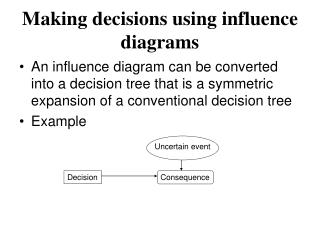DownloadDownload PresentationMaking decisions using influence diagrams

# Making decisions using influence diagrams

Download Presentation## Making decisions using influence diagrams

- - - - - - - - - - - - - - - - - - - - - - - - - - - E N D - - - - - - - - - - - - - - - - - - - - - - - - - - -
##### Presentation Transcript

1. Making decisions using influence diagrams • An influence diagram can be converted into a decision tree that is a symmetric expansion of a conventional decision tree • Example Uncertain event Decision Consequence

2. Consequence table

3. Symmetric decision tree Sunshine (p) Take Rain (1-p) Sunshine (p) Do not take Rain (1-p)

4. Procedure for making decisions • Find chance nodes that can be replaced by expected value of payoff of uncertain event (e.g., weather) • Directly precede consequence node • Do not directly precede other node • Find decision that can be replaced by branch with optimum expected value (e.g., take or not take umbrella)

5. Example Uncertain event Decision Consequence Uncertain event Decision Consequence Action Consequence Take 0.8 Do not take p Solution: Take umbrella if Pr(sunshine)<0.8, otherwise do not take umbrella

6. Example: Imperfect information Original influence diagram Forecast Path Consequence Wait for forecast? Evacuate? Stay Evacuate Stay Evacuate

7. Challenge • There is no chance node to reduce • Path of storm affects forecast • Solution: consider that the forecast is the fundamental uncertainty that influences the path of the storm. In terms of the influence diagram, reverse the arrow from the path to the forecast. • Need probabilities of forecasting hit (H’) and miss (M’) and conditional probabilities of hit and miss given that the forecast is hit, and miss. • Find these probabilities using Bayes’ rule.

8. Modified influence diagram Forecast Path Consequence Wait for forecast? Evacuate? Stay Evacuate Stay Evacuate

9. Bayes’ rule • Find probability of a hypothesis being true given the evidence, P(H/E) • Usually it is easier to estimate probability of getting evidence E given that the hypothesis is true, P(E/H)

10. Example • One 4-sided die, one 20-sided die • Prior probabilities of picking the 4-sided and 20-sided = 0.5 • Evidence: One die was picked at random. We do not know which die was picked. We rolled the die once and got 3. • What is the updated probability of each die in the light of evidence?

11. Prior information Evidence, E: 3 4-sided P=0.5 4-sided P=0.5 20-sided P=0.5 20-sided P=0.5 Example P(E/4-sided)=0.25 P(E/20-sided)=0.05

12. Example • Hypothesis, H: 4-sided die was selected • P(E/H)=P(3/4-sided) = 0.25 • P(E/HC)=P(3/20-sided) = 0.05 • Posterior obtained from Bayes theorem.

13. ExamplePosterior Probabilities 4-sided P=0.833 20-sided P=0.167

14. Modified influence diagram Forecast Path Consequence Wait for forecast? Evacuate? Stay Evacuate Stay Evacuate

15. Consequence matrix

16. Computing probabilities of the storm hitting and missing Miami given that the forecast says so

17. Computing probabilities of the storm hitting and missing Miami given that the forecast says it will miss it

18. First step in solving influence diagram Forecast Consequence Wait for forecast? Evacuate? Stay Evacuate Stay Evacuate

19. If forecast=hit Miami, evacuate. Otherwise stay.

20. Second step in solving influence diagram Forecast Wait for forecast? Consequence Stay Evacuate

21. Third step in solving influence diagram Wait for forecast? Consequence Stay Evacuate

22. Solution: Stay and wait for forecast. If forecast says storm will hit Miami, evacuate. Otherwise stay. Value of additional information from forecast=0.874-0.8=0.074